Durer: Drawing of a pentagon

The first time I read about this amazing construction of a non-regular pentagon by Dürer was in Dan Pedoe's book 'Geometry and the Liberal Arts' (an Spanish version was published by Gustavo Gili Editores in 1979).

As Pedoe pointed out 'Dürer's interest in the construction of regular polygons is a reflection of their use in the Middle Ages in both Islamic and Gothic decoration and, after the invention of firearms, in the construction of fortifications.' (Pedoe, p. 68)

In Dürer's book 'Underweysung der Messung' ('Four Books of Measurement', published in 1525) the author gives an exact construction for a regular pentagon (taken from Ptolemy) but here we are playing with another construction of a pentagon, in this case is an equilateral but not equiangular pentagon.

Dürer thought that 'geometry is the right foundation of all painting' and he wanted to be accessible to painters, artists in general and craftsmen. Then he wrote his books in German and the his focus was practical. The construction that we are studying now is a good example because, although it is approximate, it is very accurate and simple to draw.

He draw it using a rusty compass (a compass with fixed radius) that makes the task easier. [Abu'l-Wafa Al-Buzjani (940-998), mathematician and astronomer, was interested in this kind of geometric constructions using a rusty compass and wrote 'A book on those geometric constructions which are necessary for a craftsman'].In this page of Dürer's book 'Underweysung der Messung' you can see two constructions of pentagons using ruler and compass: one is exact and the other is approximate.This is the approximate construction of an equilatera but not equiangular pentagon. (This construction was well known by craftsman in Durer's time and was publishen in the book 'Geometria Deutsch', a workshop manual, around 1484)

We already know that the five angles of a regular pentagon are 108º. Pedoe wrote that, in Dürer's approximate construction, the angles in the base are 108º21'58'', there are two angles less than 108º and the angle on the top is larger than 109º. This differences would hardly be detectable in a drawing.

We are going to use trigonometry to calculate these angles. For simplicity we can consider that que side length is 1.You can start drawing angle ABF: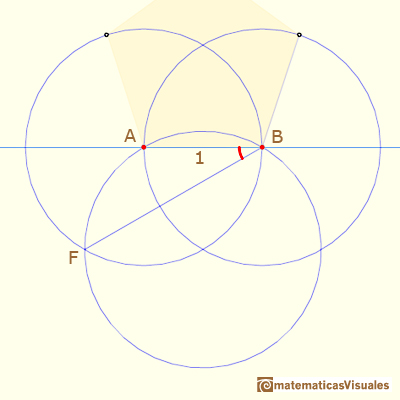The distance between B and F is twice the height of an equilateral triangle: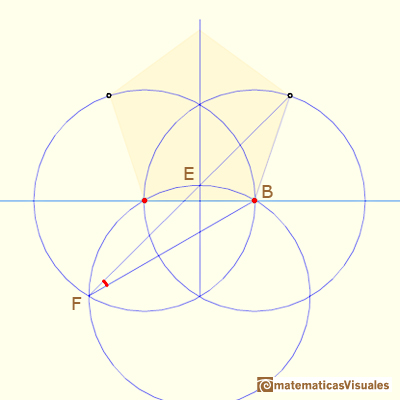If you remember the properties of the central and inscribed angles in a circle, this is very easy:

Using the Law of Sines we can calculate angle FHB:Now we can calculate angle ABH: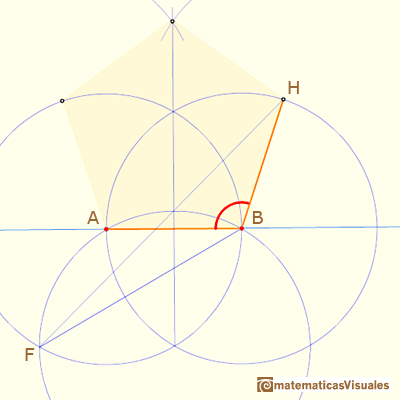And this angle is bigger than 108º, but the error is very small.

To calculate angles HKI and BHK we can start calculating BZ:If M is the point in the middle between H and I:We can calculate angle MHK:Then, the triangle IHK is an isosceles triangle and it is very easy to calculate angle HKI:This angle is bigger than 108º.

Finishing our job is now an easy task. Remember that the five angles of a convex pentagon measure three times 180º. We can deduce angle BHKThe angle at the top is smaller than 108º.

We can play with the zoom and see how accurate is Durer's approximation:

Even zooming you can see that the approximation is very good.Very close we can see the small error:The height of Dürer's pentagon is smaller than the height of a regular pentagon and the angle at the top is bigger:REFERENCES

Albrecht Durer's Underweysung der Messung in SLUB Dresden, Digital Collection.
Dan Pedoe, Geometry and the Visual Arts. Dover Publications. (pp. 66-73)
Donald W. Crowe, Albrecht Dürer and the regular pentagon, in Fivefolded Symmetry by Istvan Hargittai Ed., World Scientific Publishing Co.
Erwin Panofsky, The Life and Art of Albrecht Dürer.The diagonal of a regular pentagon are in golden ratio to its sides and the point of intersection of two diagonals of a regular pentagon are said to divide each other in the golden ratio or 'in extreme and mean ratio'.From Euclid's definition of the division of a segment into its extreme and mean ratio we introduce a property of golden rectangles and we deduce the equation and the value of the golden ratio.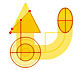Durer was the first who published in german a method to draw ellipses as cone sections.Durer made a mistake when he explanined how to draw ellipses. We can prove, using only basic properties, that the ellipse has not an egg shape .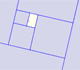A golden rectangle is made of an square and another golden rectangle.A golden rectangle is made of an square an another golden rectangle. These rectangles are related through an dilative rotation.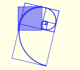The golden spiral is a good approximation of an equiangular spiral.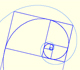Two equiangular spirals contains all vertices of golden rectangles.The twelve vertices of an icosahedron lie in three golden rectangles. Then we can calculate the volume of an icosahedronSome properties of this platonic solid and how it is related to the golden ratio. Constructing dodecahedra using different techniques.The first drawing of a plane net of a regular dodecahedron was published by Dürer in his book 'Underweysung der Messung' ('Four Books of Measurement'), published in 1525 .Leonardo da Vinci made several drawings of polyhedra for Luca Pacioli's book 'De divina proportione'. Here we can see an adaptation of the dodecahedron.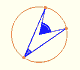Central angle in a circle is twice the angle inscribed in the circle.Using a ruler and a compass we can draw fifteen degrees angles. These are basic examples of the central and inscribed in a circle angles property.